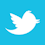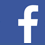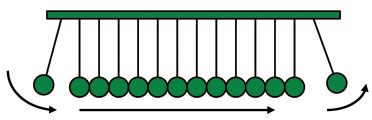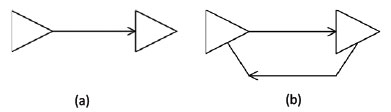Some engineers claim electrical current is not the flow of electrons. They are wrong.

Current is defined as the flow of electrons.1 The classic definition of one amp of current is one coulomb of charge flowing across a cross-sectional area in one second of time. And one coulomb of charge is defined as the charge on 6.25 x 1018 electrons. It’s a little hard to pin down the actual date of the definition of current, but it is probably around the early 1800s. That was about 200 years ago.

Certain engineers in our industry argue this definition is obsolete and that (electrical) current is not the flow of electrons. They argue it is actually a wave phenomenon. They point primarily to the speed of electron flow compared to the speed of the signal to prove their point. The speed of electron flow (defined as the drift velocity) is quite slow. Most of us can walk faster than that. The speed of signal flow down a conductor is at the speed of light (they say). Therefore, current can’t be the flow of electrons.

Here are some of the problems with that argument:

1. The word “flow” in the definition is probably a poor choice. A better word might be “movement.” As I will show, current is the movement of electrons.
2. The word “wave” in the argument is misapplied. As I will show, the correct phenomenon is a transfer of energy.
3. Signals down a conductor don’t travel at the speed of light. Neither the electrons, the conductive material (copper) nor the “wave” (transfer of energy) dictates the speed of the signal. Something else entirely dictates the speed of the signal, which is usually slower than the speed of light.

Spoiler alert: If there is a changing (AC) signal current propagating in a conductor, there is a movement of electrons within the conductor and an electromagnetic field around the conductor, each intimately associated with the other. There is a strong, symbiotic relationship that exists between them, and it is this relationship that is important.

Important Laws

Before we start the discussion, I’d like to highlight a couple of important laws. These will help in our understanding.

1. Coulomb’s Law (1785): There are two kinds of charge, positive and negative. Like charges repel, unlike attract, with force proportional to the product of their charge and inversely proportional to the square of their distance. (When two charges are very close, this coulomb force can be very strong.)
2. Ampere’s Law (1825): An electric current is accompanied by a magnetic field whose direction is at right angles to the current flow. (Therefore, a changing current is accompanied by a changing magnetic field.)
3. Faraday’s Law of Magnetic Induction (1831): A changing magnetic field is accompanied by a changing electric field at right angles to the change of the magnetic field.
4. Current must flow in a closed loop. (Corollary: therefore, every signal has a return.)

Inside the Conductor

Copper, silver and gold have the lowest resistivities of all the elements in the Periodic Table. They are known as good conductors, and they each have two very important properties in common: They are solids at room temperature and have single electrons in their outer, or valence, shells (or “bands”). Elements with single electrons in their outer shell are typically good conductors of electricity. These single electrons are not tightly held to their parent atoms. In fact, it is not always clear which atom “owns” which of these “outer” electrons. They are often (inappropriately) referred to as “free” electrons. As an electrical force (Coulomb’s Law) is applied to a conductor,2 electrons tend to “jump” from one atom to the next (FIGURE 1), displacing that atom’s electron. This constitutes the “flow” or movement of electrons.Figure 1. Single electrons in the outer, or valence, shell are free to move among similar atoms.

There are orders of magnitude more electrons “available” in a cross-sectional area of a copper conductor than are necessary for carrying the current. We can think of each “available” electron participating in the current flow for only a very small fraction of the time the current is flowing. Indeed, the fraction of available electrons actually involved in the current at any point in time is directly related to the heating of the conductor. That is why smaller conductors get hotter for the same current flow than do larger conductors.

If we look at any single electron in Figure 1, it moves under the applied force (Coulomb’s Law) from one location to another, transfers its energy to another electron, and then stays there (or perhaps gets deflected in a different direction) until another electron transfers its energy to it. All these individual reflections are pretty chaotic and happen in all directions. But there is a strong, overall trend in the “forward” direction as a result of the electric field caused by the external coulomb force.

So the rate at which any individual electron moves along the conductor is pretty slow. But what is happening is suggested better in FIGURE 2.Figure 2. Electron flow causes a shift in electrons along a conductor.

We are all familiar with this desktop toy shown in Figure 2. The falling ball at the front hits the first ball and transfers its energy to it, which then transfers energy to the second ball, and so on. The last ball in the string pops out (in the absence of friction) as far as the first ball fell. We could think of this string of balls as being very long. Even so, in the absence of friction, the last ball would pop out almost instantaneously, (almost) at the speed of light, even though the first ball is not moving very fast at all. This is a rough analogy of what happens with electrons. And the distinction is very important.

Current “flow” is really kind of a misnomer. It does not mean an electron is flowing through the conductor from beginning to end. It does not mean “one electron in, same electron out.” Instead, it means for every electron that enters the conductor, another electron must leave the conductor. What we have is “one electron in, another electron out.” This can happen almost instantaneously, as Figure 2 suggests. (Note: If “one electron in, one electron out” were not the case, electrons would collect in the conductor or drain out of the conductor, neither of which can happen.)

What is really happening in the conductor is a transfer of energy from one electron to another. How that happens can be interpreted different ways. It might occur because of the forces behind Coulomb’s Law. It might happen because of photons being emitted. For our purposes, it doesn’t matter how it happens. What happens is that when an electron is somehow transferred into a conductor at one end, another electron must exit the conductor at the other end, and does so at (almost) the speed of light.

This is different from a “wave.” A wave implies a flow of energy that does not involve a particle. A wave implies the energy would pass by the atoms and their electrons as it propagates along the conductor. But the transfer of energy here intimately involves particles (electrons), as the energy is discretely transferred from one particle to another.

Now current can only flow in a closed loop. Consider a driver connected by a trace to a receiver, as suggested in FIGURE 3(a). No current can flow in the trace or conductor. Only when there is a return loop, as shown in Figure 3(b), can current flow.Figure 3. Current can only flow in a closed loop (b). No current flows in (a).

A wave doesn’t necessarily require a physical conductor return loop. For example, a single wire transmitting antenna doesn’t have a physical conductor return loop (although there is a capacitive return loop, sometimes through the air). But an electrical current does require a physical return loop.

Consider a hairline crack in a copper trace on a circuit board. Make the hairline crack as narrow as you want. The current will not flow across the crack, even at very high frequencies. If current were a wave, we can imagine that the wave should be able to “jump” over the crack (waves can presumably travel through the air), but we know (some of us from actual experience) that the current will not jump a physical crack. One might argue some sort of capacitive action might take place, but this argument will go nowhere. We have enough experience with capacitive self-resonant frequencies, and the very small capacitances involved, that this situation would look more like a simple circuit with a very small series capacitor than a pure conductor with a perturbation too small to be seen by a wave.

Outside the Conductor

When a current starts to flow along a conductor, by Ampere’s Law a magnetic field is generated around the conductor. This is the principle behind an electromagnet. Many of us saw an experiment in elementary or high school where a wire connecting the ends of a battery was routed near a compass. The compass needle moved! Intuitively, then, a changing current in a conductor creates a changing magnetic field around the conductor. It takes energy to create this magnetic field. Energy is power times time, and in the absence of any losses, 100% of this energy is stored in the magnetic field. If the current flow stops, the field collapses, and the energy is returned to the conductor.

Now by Faraday’s Law of Magnetic Induction, a changing magnetic field creates a changing electrical field. This is the principle behind a transformer and a motor or generator.

Collectively, these two fields are called the electromagnetic field. Electromagnetic fields exist around any conductor carrying an AC current (or even just a changing current). As board designers, we are aware we need to worry about the possible negative effects of these fields (EMI, crosstalk, etc.), and we make design decisions accordingly.

Perhaps when people refer to current being a “wave” phenomenon, they are referring to the electromagnetic wave. But if you were following the argument above, you noted the current comes first! The current generates the electromagnetic field. If the current stops, the field generation stops, and the field collapses.3
We don’t get an electromagnetic field unless we have a current. The two things – the electromagnetic field and current – are intimately connected. You can’t have one without the other. So, the electromagnetic wave is not the (signal) current; the wave is generated by the (signal) current.

Not only that, but the components cannot be separated. You can’t have a changing current without a changing magnetic field. You can’t have a changing magnetic field without a changing electric field. You can’t have changing fields without a changing current (at least in any practical sense).

And further still, no one of the components can get “out in front” of the others. They all propagate down the conductor at the same speed. The electromagnet wave doesn’t travel faster than the current, and the current doesn’t travel faster than the wave. Neither component of the electromagnetic wave can get out in front of the other. They all travel at the same speed.

And how is that speed determined for a signal in a conductor? It is not determined by the electrons. It is not determined by the copper. The speed of the signal is determined by the speed of the electromagnetic field (around the conductor) in the material itflows through (the dielectric.) If the conductor is a bare wire in air, the propagation speed will approach the speed of light, about 1.0 ft./ns. But if the conductor is surrounded by a dielectric, so that the electromagnetic field travels though the dielectric (as in the case of a stripline stackup, for example), then the propagation speed is reduced by the square root of the relative dielectric coefficient of the board material.

The Combined System

So when we send a signal current down a trace:

1. There must be a continuous conductive loop for the signal current to travel in.
2. Any charged particle (e.g., electron) “injected” in the beginning of the circuit will be accompanied by an identical charged particle leaving the circuit at the other end of the circuit.
3. The signal current will consist of a transfer of energy among charged particles (electrons) in the conductor.
4. An electromagnetic waveform will be generated around the conductor and will travel along the conductor.
5. The propagation speed of the signal will be determined by the environment the electromagnetic field flows through.

So, while there is a(n electromagnetic) field around the conductor, the definition of current is the movement of electrons through the conductor, driven typically by coulomb forces. And, in good healthy synergy, we note the current flows though the conductor, while the energy flows in the electromagnetic field around the conductor. This is the symbiotic relationship that exists between the current and the electromagnetic field.

Notes
1. See sidebar for several different sources of this definition, including a quote by Nobel Prize-winning physicist Richard Feynman.
2. Attaching a battery is one way of applying a coulomb force across a conductor.
3. It is important to recognize that current and the generation of the electromagnetic field are “point” phenomena. That is, the current exists at a point along the conductor and may have different levels at different points. So the current at a point generates a field at a point, and then the two components travel along the path together.

Bibliography

1. Douglas Brooks, PCB Currents: How They Flow, How They React, Prentice Hall, 2017.
2. Douglas Brooks, Maxwell’s Equations without the Calculus, 2016, amazon.com.

Douglas Brooks, Ph.D., is president of UltraCAD Design, a PCB design service bureau and author of three books, including PCB Trace and Via Currents and Temperatures: The Complete Analysis, 2nd edition (with Johannes Adam) and PCB Currents: How They Flow, How They React; This email address is being protected from spambots. You need JavaScript enabled to view it..

A Sampling of Definitions

A search for “definition of electric current” produces an endless number of hits. Here is a sample:

• The time rate of flow of electric charge (dictionary.com/browse/electric-current)
• An electric current is a flow of electric charge. In electric circuits this charge is often carried by moving electrons in a wire. It can also be carried by ions in an electrolyte. (en.wikipedia.org/wiki/Electric_current)
• Electric current is defined as the rate at which charge flows through a surface (the cross section of a wire, for example). (physics.info/electric-current)
• An ampere is a unit of measure of the rate of electron flow or current in an electrical conductor. One ampere of current represents one coulomb of electrical charge (6.24 x 10^18 charge carriers) moving past a specific point in one second.(whatis.techtarget.com/definition/ampere)
• And from no less than Nobel Prize-winning physicist Richard Feynman:

In an atom with three protons in the nucleus exchanging photons with three electrons – a condition called a lithium atom – the third electron is further away from the nucleus than the other two (which have used up the available space), and exchanges fewer photons. This causes the electron to easily break away from its own nucleus under the influence of photons from other atoms. A large number of such atoms close together easily lose their individual third electrons to form a sea of electrons swimming around from atom to atom. This sea of electrons reacts to any small electrical force (photons), generating a current of electrons – I am describing lithium metal conducting electricity. Hydrogen and helium atoms do not lose their electrons to other atoms. They are “insulators.” (Richard P. Feynman, QED, The Strange Theory of Light and Matter, Princeton Scientific Library, 1985.)